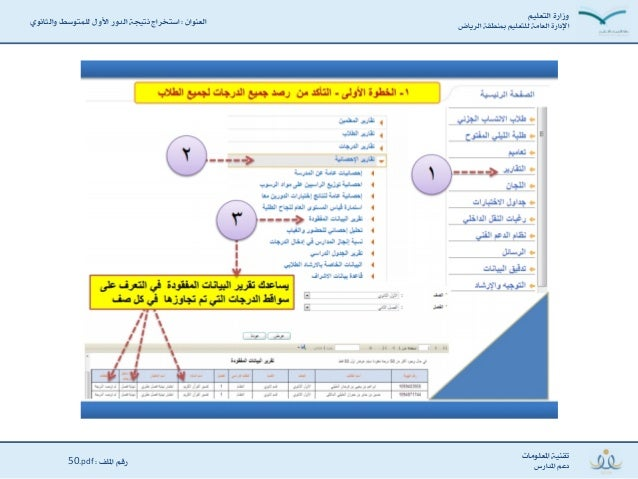Successfully reported this slideshow.Upcoming SlideShare
×

استخراج نتيجة الدور الأول للمتوسط والثانوي

596,749 views

Published on

استخراج نتيجة الدور الأول للمتوسط والثانوي

Published in: Education
• Full Name
Comment goes here.

Are you sure you want to Yes No• Be the first to comment

• Be the first to like this

استخراج نتيجة الدور الأول للمتوسط والثانوي

1. 1. ‹È◊√j÷]<ÏÖ]áÊ< <ÏÖ]Å˝]<‹È◊√j◊÷<Ì⁄^√÷]ö^ËÜ÷]<Ìœ�ﬂ≤<<·]Áﬂ√÷]<V<ºâÁj€◊÷<ŸÊ˘]<ÖÊÇ÷]<ÌrÈjﬁ<t]Ü~jâ]ÎÁﬁ^n÷]Ê< l^⁄Á◊√π]<ÌÈﬂœi< åÖ]Çπ]<‹¬Å<<Ã◊π]<‹ŒÖ<V50.pdf<
2. 2. l^⁄Á◊√π]<ÌÈﬂœi< åÖ]Çπ]<‹¬Å<<Ã◊π]<‹ŒÖ<V50.pdf< ‹È◊√j÷]<ÏÖ]áÊ< <ÏÖ]Å˝]<‹È◊√j◊÷<Ì⁄^√÷]ö^ËÜ÷]<Ìœ�ﬂ≤<<·]Áﬂ√÷]<V<ºâÁj€◊÷<ŸÊ˘]<ÖÊÇ÷]<ÌrÈjﬁ<t]Ü~jâ]ÎÁﬁ^n÷]Ê<
3. 3. l^⁄Á◊√π]<ÌÈﬂœi< åÖ]Çπ]<‹¬Å<<Ã◊π]<‹ŒÖ<V50.pdf< ‹È◊√j÷]<ÏÖ]áÊ< <ÏÖ]Å˝]<‹È◊√j◊÷<Ì⁄^√÷]ö^ËÜ÷]<Ìœ�ﬂ≤<<·]Áﬂ√÷]<V<ºâÁj€◊÷<ŸÊ˘]<ÖÊÇ÷]<ÌrÈjﬁ<t]Ü~jâ]ÎÁﬁ^n÷]Ê<
4. 4. l^⁄Á◊√π]<ÌÈﬂœi< åÖ]Çπ]<‹¬Å<<Ã◊π]<‹ŒÖ<V50.pdf< ‹È◊√j÷]<ÏÖ]áÊ< <ÏÖ]Å˝]<‹È◊√j◊÷<Ì⁄^√÷]ö^ËÜ÷]<Ìœ�ﬂ≤<<·]Áﬂ√÷]<V<ºâÁj€◊÷<ŸÊ˘]<ÖÊÇ÷]<ÌrÈjﬁ<t]Ü~jâ]ÎÁﬁ^n÷]Ê<
5. 5. l^⁄Á◊√π]<ÌÈﬂœi< åÖ]Çπ]<‹¬Å<<Ã◊π]<‹ŒÖ<V50.pdf< ‹È◊√j÷]<ÏÖ]áÊ< <ÏÖ]Å˝]<‹È◊√j◊÷<Ì⁄^√÷]ö^ËÜ÷]<Ìœ�ﬂ≤<<·]Áﬂ√÷]<V<ºâÁj€◊÷<ŸÊ˘]<ÖÊÇ÷]<ÌrÈjﬁ<t]Ü~jâ]ÎÁﬁ^n÷]Ê<
6. 6. l^⁄Á◊√π]<ÌÈﬂœi< åÖ]Çπ]<‹¬Å<<Ã◊π]<‹ŒÖ<V50.pdf< ‹È◊√j÷]<ÏÖ]áÊ< <ÏÖ]Å˝]<‹È◊√j◊÷<Ì⁄^√÷]ö^ËÜ÷]<Ìœ�ﬂ≤<<·]Áﬂ√÷]<V<ºâÁj€◊÷<ŸÊ˘]<ÖÊÇ÷]<ÌrÈjﬁ<t]Ü~jâ]ÎÁﬁ^n÷]Ê<
7. 7. l^⁄Á◊√π]<ÌÈﬂœi< åÖ]Çπ]<‹¬Å<<Ã◊π]<‹ŒÖ<V50.pdf< ‹È◊√j÷]<ÏÖ]áÊ< <ÏÖ]Å˝]<‹È◊√j◊÷<Ì⁄^√÷]ö^ËÜ÷]<Ìœ�ﬂ≤<<·]Áﬂ√÷]<V<ºâÁj€◊÷<ŸÊ˘]<ÖÊÇ÷]<ÌrÈjﬁ<t]Ü~jâ]ÎÁﬁ^n÷]Ê<
8. 8. l^⁄Á◊√π]<ÌÈﬂœi< åÖ]Çπ]<‹¬Å<<Ã◊π]<‹ŒÖ<V50.pdf< ‹È◊√j÷]<ÏÖ]áÊ< <ÏÖ]Å˝]<‹È◊√j◊÷<Ì⁄^√÷]ö^ËÜ÷]<Ìœ�ﬂ≤<<·]Áﬂ√÷]<V<ºâÁj€◊÷<ŸÊ˘]<ÖÊÇ÷]<ÌrÈjﬁ<t]Ü~jâ]ÎÁﬁ^n÷]Ê<
9. 9. l^⁄Á◊√π]<ÌÈﬂœi< åÖ]Çπ]<‹¬Å<<Ã◊π]<‹ŒÖ<V50.pdf< ‹È◊√j÷]<ÏÖ]áÊ< <ÏÖ]Å˝]<‹È◊√j◊÷<Ì⁄^√÷]ö^ËÜ÷]<Ìœ�ﬂ≤<<·]Áﬂ√÷]<V<ºâÁj€◊÷<ŸÊ˘]<ÖÊÇ÷]<ÌrÈjﬁ<t]Ü~jâ]ÎÁﬁ^n÷]Ê<
10. 10. l^⁄Á◊√π]<ÌÈﬂœi< åÖ]Çπ]<‹¬Å<<Ã◊π]<‹ŒÖ<V50.pdf< ‹È◊√j÷]<ÏÖ]áÊ< <ÏÖ]Å˝]<‹È◊√j◊÷<Ì⁄^√÷]ö^ËÜ÷]<Ìœ�ﬂ≤<<·]Áﬂ√÷]<V<ºâÁj€◊÷<ŸÊ˘]<ÖÊÇ÷]<ÌrÈjﬁ<t]Ü~jâ]ÎÁﬁ^n÷]Ê<
11. 11. l^⁄Á◊√π]<ÌÈﬂœi< åÖ]Çπ]<‹¬Å<<Ã◊π]<‹ŒÖ<V50.pdf< ‹È◊√j÷]<ÏÖ]áÊ< <ÏÖ]Å˝]<‹È◊√j◊÷<Ì⁄^√÷]ö^ËÜ÷]<Ìœ�ﬂ≤<<·]Áﬂ√÷]<V<ºâÁj€◊÷<ŸÊ˘]<ÖÊÇ÷]<ÌrÈjﬁ<t]Ü~jâ]ÎÁﬁ^n÷]Ê<
12. 12. l^⁄Á◊√π]<ÌÈﬂœi< åÖ]Çπ]<‹¬Å<<Ã◊π]<‹ŒÖ<V50.pdf< ‹È◊√j÷]<ÏÖ]áÊ< <ÏÖ]Å˝]<‹È◊√j◊÷<Ì⁄^√÷]ö^ËÜ÷]<Ìœ�ﬂ≤<<·]Áﬂ√÷]<V<ºâÁj€◊÷<ŸÊ˘]<ÖÊÇ÷]<ÌrÈjﬁ<t]Ü~jâ]ÎÁﬁ^n÷]Ê<
13. 13. l^⁄Á◊√π]<ÌÈﬂœi< åÖ]Çπ]<‹¬Å<<Ã◊π]<‹ŒÖ<V50.pdf< ‹È◊√j÷]<ÏÖ]áÊ< <ÏÖ]Å˝]<‹È◊√j◊÷<Ì⁄^√÷]ö^ËÜ÷]<Ìœ�ﬂ≤<<·]Áﬂ√÷]<V<ºâÁj€◊÷<ŸÊ˘]<ÖÊÇ÷]<ÌrÈjﬁ<t]Ü~jâ]ÎÁﬁ^n÷]Ê<
14. 14. l^⁄Á◊√π]<ÌÈﬂœi< åÖ]Çπ]<‹¬Å<<Ã◊π]<‹ŒÖ<V50.pdf< ‹È◊√j÷]<ÏÖ]áÊ< <ÏÖ]Å˝]<‹È◊√j◊÷<Ì⁄^√÷]ö^ËÜ÷]<Ìœ�ﬂ≤<<·]Áﬂ√÷]<V<ºâÁj€◊÷<ŸÊ˘]<ÖÊÇ÷]<ÌrÈjﬁ<t]Ü~jâ]ÎÁﬁ^n÷]Ê<
15. 15. l^⁄Á◊√π]<ÌÈﬂœi< åÖ]Çπ]<‹¬Å<<Ã◊π]<‹ŒÖ<V50.pdf< ‹È◊√j÷]<ÏÖ]áÊ< <ÏÖ]Å˝]<‹È◊√j◊÷<Ì⁄^√÷]ö^ËÜ÷]<Ìœ�ﬂ≤<<·]Áﬂ√÷]<V<ºâÁj€◊÷<ŸÊ˘]<ÖÊÇ÷]<ÌrÈjﬁ<t]Ü~jâ]ÎÁﬁ^n÷]Ê<
16. 16. l^⁄Á◊√π]<ÌÈﬂœi< åÖ]Çπ]<‹¬Å<<Ã◊π]<‹ŒÖ<V50.pdf< ‹È◊√j÷]<ÏÖ]áÊ< <ÏÖ]Å˝]<‹È◊√j◊÷<Ì⁄^√÷]ö^ËÜ÷]<Ìœ�ﬂ≤<<·]Áﬂ√÷]<V<ºâÁj€◊÷<ŸÊ˘]<ÖÊÇ÷]<ÌrÈjﬁ<t]Ü~jâ]ÎÁﬁ^n÷]Ê<
17. 17. l^⁄Á◊√π]<ÌÈﬂœi< åÖ]Çπ]<‹¬Å<<Ã◊π]<‹ŒÖ<V50.pdf< ‹È◊√j÷]<ÏÖ]áÊ< <ÏÖ]Å˝]<‹È◊√j◊÷<Ì⁄^√÷]ö^ËÜ÷]<Ìœ�ﬂ≤<<·]Áﬂ√÷]<V<ºâÁj€◊÷<ŸÊ˘]<ÖÊÇ÷]<ÌrÈjﬁ<t]Ü~jâ]ÎÁﬁ^n÷]Ê<
18. 18. l^⁄Á◊√π]<ÌÈﬂœi< åÖ]Çπ]<‹¬Å<<Ã◊π]<‹ŒÖ<V50.pdf< ‹È◊√j÷]<ÏÖ]áÊ< <ÏÖ]Å˝]<‹È◊√j◊÷<Ì⁄^√÷]ö^ËÜ÷]<Ìœ�ﬂ≤<<·]Áﬂ√÷]<V<ºâÁj€◊÷<ŸÊ˘]<ÖÊÇ÷]<ÌrÈjﬁ<t]Ü~jâ]ÎÁﬁ^n÷]Ê<